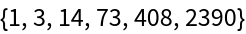Function Repository Resource:

# IrreducibleBinaryCompositions

List all irreducible binary compositions for a set of chosen symbols and a chosen simplification rule

 ResourceFunction["IrreducibleBinaryCompositions"][rules,n,sym] returns a rules-irreducible subset of all possible binary compositions of symbols listed in sym whose proper leaf count (terminal nodes only) equals n. ResourceFunction["IrreducibleBinaryCompositions"][rules,n] assumes compositions of only two formal variables, a and b.

## Details

The argument rules is assumed to be one or more simplification rules; i.e. rules that reduce the leaf count.
The subset excludes compositions which match the left hand side of the rules on a subexpression at any level, and includes all other non-matching compositions.

## Examples

### Basic Examples (1)

Generate binary compositions where a never appears as a left argument:

 In:=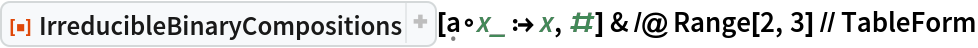Out=### Scope (3)

Use more than one rule to allow for quantitatively different reduction paths:

 In:=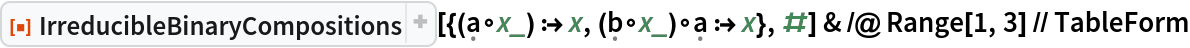Out=For some choices of rule binary compositions are totally reducible:

 In:=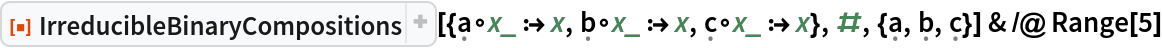Out=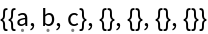The following count of irreducible compositions is related to the super-Catalan numbers (OEIS A001003):

 In:=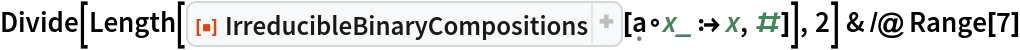Out=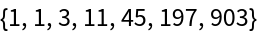### Neat Examples (2)

Plot irreducible expressions as trees for a choice of leaf count equals three:

 In:=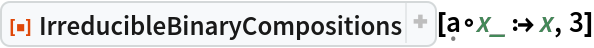Out=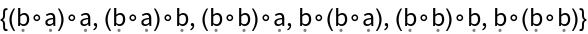In:=Out=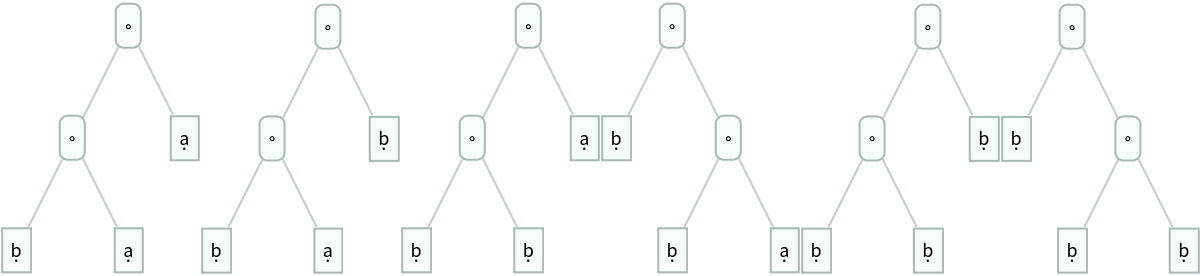A sequence that does not appear in the OEIS:

 In:=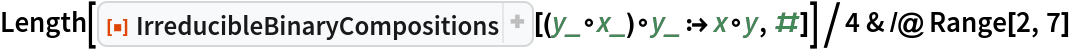Out=